You are reading an old version of the documentation (v2.0.0). For the latest version see https://matplotlib.org/stable/api/mlab_api.htmlTravis-CI:# mlab¶

## `matplotlib.mlab`¶

Numerical python functions written for compatability with MATLAB commands with the same names.

### MATLAB compatible functions¶

`cohere()`
Coherence (normalized cross spectral density)
`csd()`
Cross spectral density using Welch’s average periodogram
`detrend()`
Remove the mean or best fit line from an array
`find()`
Return the indices where some condition is true; numpy.nonzero is similar but more general.
`griddata()`
Interpolate irregularly distributed data to a regular grid.
`prctile()`
Find the percentiles of a sequence
`prepca()`
Principal Component Analysis
`psd()`
Power spectral density using Welch’s average periodogram
`rk4()`
A 4th order runge kutta integrator for 1D or ND systems
`specgram()`
Spectrogram (spectrum over segments of time)

### Miscellaneous functions¶

Functions that don’t exist in MATLAB, but are useful anyway:

`cohere_pairs()`
Coherence over all pairs. This is not a MATLAB function, but we compute coherence a lot in my lab, and we compute it for a lot of pairs. This function is optimized to do this efficiently by caching the direct FFTs.
`rk4()`
A 4th order Runge-Kutta ODE integrator in case you ever find yourself stranded without scipy (and the far superior scipy.integrate tools)
`contiguous_regions()`
Return the indices of the regions spanned by some logical mask
`cross_from_below()`
Return the indices where a 1D array crosses a threshold from below
`cross_from_above()`
Return the indices where a 1D array crosses a threshold from above
`complex_spectrum()`
Return the complex-valued frequency spectrum of a signal
`magnitude_spectrum()`
Return the magnitude of the frequency spectrum of a signal
`angle_spectrum()`
Return the angle (wrapped phase) of the frequency spectrum of a signal
`phase_spectrum()`
Return the phase (unwrapped angle) of the frequency spectrum of a signal
`detrend_mean()`
Remove the mean from a line.
`demean()`
Remove the mean from a line. This function is the same as `detrend_mean()` except for the default axis.
`detrend_linear()`
Remove the best fit line from a line.
`detrend_none()`
Return the original line.
`stride_windows()`
Get all windows in an array in a memory-efficient manner
`stride_repeat()`
Repeat an array in a memory-efficient manner
`apply_window()`
Apply a window along a given axis

### record array helper functions¶

A collection of helper methods for numpyrecord arrays

`rec2txt()`
Pretty print a record array
`rec2csv()`
Store record array in CSV file
`csv2rec()`
Import record array from CSV file with type inspection
`rec_append_fields()`
Adds field(s)/array(s) to record array
`rec_drop_fields()`
Drop fields from record array
`rec_join()`
Join two record arrays on sequence of fields
`recs_join()`
A simple join of multiple recarrays using a single column as a key
`rec_groupby()`
Summarize data by groups (similar to SQL GROUP BY)
`rec_summarize()`
Helper code to filter rec array fields into new fields

For the rec viewer functions(e rec2csv), there are a bunch of Format objects you can pass into the functions that will do things like color negative values red, set percent formatting and scaling, etc.

Example usage:

```r = csv2rec('somefile.csv', checkrows=0)

formatd = dict(
weight = FormatFloat(2),
change = FormatPercent(2),
cost   = FormatThousands(2),
)

rec2excel(r, 'test.xls', formatd=formatd)
rec2csv(r, 'test.csv', formatd=formatd)
scroll = rec2gtk(r, formatd=formatd)

win = gtk.Window()
win.set_size_request(600,800)
win.show_all()
gtk.main()
```
class `matplotlib.mlab.``FormatBool`
`fromstr`(s)
`toval`(x)
class `matplotlib.mlab.``FormatDate`(fmt)
`fromstr`(x)
`toval`(x)
class `matplotlib.mlab.``FormatDatetime`(fmt='%Y-%m-%d %H:%M:%S')
`fromstr`(x)
class `matplotlib.mlab.``FormatFloat`(precision=4, scale=1.0)
`fromstr`(s)
`toval`(x)
class `matplotlib.mlab.``FormatFormatStr`(fmt)
`tostr`(x)
class `matplotlib.mlab.``FormatInt`
`fromstr`(s)
`tostr`(x)
`toval`(x)
class `matplotlib.mlab.``FormatMillions`(precision=4)
class `matplotlib.mlab.``FormatObj`

Bases: `object`

`fromstr`(s)
`tostr`(x)
`toval`(x)
class `matplotlib.mlab.``FormatPercent`(precision=4)
class `matplotlib.mlab.``FormatString`
`tostr`(x)
class `matplotlib.mlab.``FormatThousands`(precision=4)
class `matplotlib.mlab.``GaussianKDE`(dataset, bw_method=None)

Bases: `object`

Representation of a kernel-density estimate using Gaussian kernels.

Parameters: dataset : array_like Datapoints to estimate from. In case of univariate data this is a 1-D array, otherwise a 2-D array with shape (# of dims, # of data). bw_method : str, scalar or callable, optional The method used to calculate the estimator bandwidth. This can be ‘scott’, ‘silverman’, a scalar constant or a callable. If a scalar, this will be used directly as `kde.factor`. If a callable, it should take a `GaussianKDE` instance as only parameter and return a scalar. If None (default), ‘scott’ is used.

Attributes

 dataset (ndarray) The dataset with which `gaussian_kde` was initialized. dim (int) Number of dimensions. num_dp (int) Number of datapoints. factor (float) The bandwidth factor, obtained from `kde.covariance_factor`, with which the covariance matrix is multiplied. covariance (ndarray) The covariance matrix of `dataset`, scaled by the calculated bandwidth (`kde.factor`). inv_cov (ndarray) The inverse of `covariance`.

Methods

 kde.evaluate(points) (ndarray) Evaluate the estimated pdf on a provided set of points. kde(points) (ndarray) Same as kde.evaluate(points)
`covariance_factor`()
`evaluate`(points)

Evaluate the estimated pdf on a set of points.

Parameters: points : (# of dimensions, # of points)-array Alternatively, a (# of dimensions,) vector can be passed in and treated as a single point. values : (# of points,)-array The values at each point. ValueError : if the dimensionality of the input points is different than the dimensionality of the KDE.
`scotts_factor`()
`silverman_factor`()
class `matplotlib.mlab.``PCA`(a, standardize=True)

Bases: `object`

compute the SVD of a and store data for PCA. Use project to project the data onto a reduced set of dimensions

Parameters: a : np.ndarray A numobservations x numdims array standardize : bool True if input data are to be standardized. If False, only centering will be carried out.

Notes

The factor loadings are in the `Wt` factor, i.e., the factor loadings for the first principal component are given by `Wt`. This row is also the first eigenvector.

Attributes

 a A centered unit sigma version of input `a`. numrows, numcols The dimensions of `a`. mu A numdims array of means of `a`. This is the vector that points to the origin of PCA space. sigma A numdims array of standard deviation of `a`. fracs The proportion of variance of each of the principal components. s The actual eigenvalues of the decomposition. Wt The weight vector for projecting a numdims point or array into PCA space. Y A projected into PCA space.
`center`(x)

center and optionally standardize the data using the mean and sigma from training set a

`project`(x, minfrac=0.0)

project x onto the principle axes, dropping any axes where fraction of variance<minfrac

`matplotlib.mlab.``amap`(function, sequence[, sequence, ...]) → array.

Works like `map()`, but it returns an array. This is just a convenient shorthand for `numpy.array(map(...))`.

`matplotlib.mlab.``angle_spectrum`(x, Fs=None, window=None, pad_to=None, sides=None)

Compute the angle of the frequency spectrum (wrapped phase spectrum) of x. Data is padded to a length of pad_to and the windowing function window is applied to the signal.

Parameters: x : 1-D array or sequence Array or sequence containing the data Fs : scalar The sampling frequency (samples per time unit). It is used to calculate the Fourier frequencies, freqs, in cycles per time unit. The default value is 2. window : callable or ndarray A function or a vector of length NFFT. To create window vectors see `window_hanning()`, `window_none()`, `numpy.blackman()`, `numpy.hamming()`, `numpy.bartlett()`, `scipy.signal()`, `scipy.signal.get_window()`, etc. The default is `window_hanning()`. If a function is passed as the argument, it must take a data segment as an argument and return the windowed version of the segment. sides : [ ‘default’ | ‘onesided’ | ‘twosided’ ] Specifies which sides of the spectrum to return. Default gives the default behavior, which returns one-sided for real data and both for complex data. ‘onesided’ forces the return of a one-sided spectrum, while ‘twosided’ forces two-sided. pad_to : integer The number of points to which the data segment is padded when performing the FFT. While not increasing the actual resolution of the spectrum (the minimum distance between resolvable peaks), this can give more points in the plot, allowing for more detail. This corresponds to the n parameter in the call to fft(). The default is None, which sets pad_to equal to the length of the input signal (i.e. no padding). spectrum : 1-D array The values for the angle spectrum in radians (real valued) freqs : 1-D array The frequencies corresponding to the elements in spectrum

`complex_spectrum()`
This function returns the angle value of `complex_spectrum()`.
`magnitude_spectrum()`
`angle_spectrum()` returns the magnitudes of the corresponding frequencies.
`phase_spectrum()`
`phase_spectrum()` returns the unwrapped version of this function.
`specgram()`
`specgram()` can return the angle spectrum of segments within the signal.
`matplotlib.mlab.``apply_window`(x, window, axis=0, return_window=None)

Apply the given window to the given 1D or 2D array along the given axis.

Parameters: x : 1D or 2D array or sequence Array or sequence containing the data. window : function or array. Either a function to generate a window or an array with length x.shape[axis] axis : integer The axis over which to do the repetition. Must be 0 or 1. The default is 0 return_window : bool If true, also return the 1D values of the window that was applied
`matplotlib.mlab.``base_repr`(number, base=2, padding=0)

Return the representation of a number in any given base.

`matplotlib.mlab.``binary_repr`(number, max_length=1025)

Return the binary representation of the input number as a string.

This is more efficient than using `base_repr()` with base 2.

Increase the value of max_length for very large numbers. Note that on 32-bit machines, 2**1023 is the largest integer power of 2 which can be converted to a Python float.

`matplotlib.mlab.``bivariate_normal`(X, Y, sigmax=1.0, sigmay=1.0, mux=0.0, muy=0.0, sigmaxy=0.0)

Bivariate Gaussian distribution for equal shape X, Y.

See bivariate normal at mathworld.

`matplotlib.mlab.``center_matrix`(M, dim=0)

Return the matrix M with each row having zero mean and unit std.

If dim = 1 operate on columns instead of rows. (dim is opposite to the numpy axis kwarg.)

`matplotlib.mlab.``cohere`(x, y, NFFT=256, Fs=2, detrend=<function detrend_none>, window=<function window_hanning>, noverlap=0, pad_to=None, sides='default', scale_by_freq=None)

The coherence between x and y. Coherence is the normalized cross spectral density: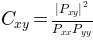Parameters: x, y Array or sequence containing the data Fs : scalar The sampling frequency (samples per time unit). It is used to calculate the Fourier frequencies, freqs, in cycles per time unit. The default value is 2. window : callable or ndarray A function or a vector of length NFFT. To create window vectors see `window_hanning()`, `window_none()`, `numpy.blackman()`, `numpy.hamming()`, `numpy.bartlett()`, `scipy.signal()`, `scipy.signal.get_window()`, etc. The default is `window_hanning()`. If a function is passed as the argument, it must take a data segment as an argument and return the windowed version of the segment. sides : [ ‘default’ | ‘onesided’ | ‘twosided’ ] Specifies which sides of the spectrum to return. Default gives the default behavior, which returns one-sided for real data and both for complex data. ‘onesided’ forces the return of a one-sided spectrum, while ‘twosided’ forces two-sided. pad_to : integer The number of points to which the data segment is padded when performing the FFT. This can be different from NFFT, which specifies the number of data points used. While not increasing the actual resolution of the spectrum (the minimum distance between resolvable peaks), this can give more points in the plot, allowing for more detail. This corresponds to the n parameter in the call to fft(). The default is None, which sets pad_to equal to NFFT NFFT : integer The number of data points used in each block for the FFT. A power 2 is most efficient. The default value is 256. This should NOT be used to get zero padding, or the scaling of the result will be incorrect. Use pad_to for this instead. detrend : {‘default’, ‘constant’, ‘mean’, ‘linear’, ‘none’} or callable The function applied to each segment before fft-ing, designed to remove the mean or linear trend. Unlike in MATLAB, where the detrend parameter is a vector, in matplotlib is it a function. The `pylab` module defines `detrend_none()`, `detrend_mean()`, and `detrend_linear()`, but you can use a custom function as well. You can also use a string to choose one of the functions. ‘default’, ‘constant’, and ‘mean’ call `detrend_mean()`. ‘linear’ calls `detrend_linear()`. ‘none’ calls `detrend_none()`. scale_by_freq : boolean, optional Specifies whether the resulting density values should be scaled by the scaling frequency, which gives density in units of Hz^-1. This allows for integration over the returned frequency values. The default is True for MATLAB compatibility. noverlap : integer The number of points of overlap between blocks. The default value is 0 (no overlap). The return value is the tuple (Cxy, f), where f are the frequencies of the coherence vector. For cohere, scaling the individual densities by the sampling frequency has no effect, since the factors cancel out.

`matplotlib.mlab.``cohere_pairs`(X, ij, NFFT=256, Fs=2, detrend=<function detrend_none>, window=<function window_hanning>, noverlap=0, preferSpeedOverMemory=True, progressCallback=<function donothing_callback>, returnPxx=False)

Compute the coherence and phase for all pairs ij, in X.

X is a numSamples * numCols array

ij is a list of tuples. Each tuple is a pair of indexes into the columns of X for which you want to compute coherence. For example, if X has 64 columns, and you want to compute all nonredundant pairs, define ij as:

```ij = []
for i in range(64):
for j in range(i+1,64):
ij.append( (i,j) )
```

preferSpeedOverMemory is an optional bool. Defaults to true. If False, limits the caching by only making one, rather than two, complex cache arrays. This is useful if memory becomes critical. Even when preferSpeedOverMemory is False, `cohere_pairs()` will still give significant performace gains over calling `cohere()` for each pair, and will use subtantially less memory than if preferSpeedOverMemory is True. In my tests with a 43000,64 array over all nonredundant pairs, preferSpeedOverMemory = True delivered a 33% performance boost on a 1.7GHZ Athlon with 512MB RAM compared with preferSpeedOverMemory = False. But both solutions were more than 10x faster than naively crunching all possible pairs through `cohere()`.

Returns: Cxy : dictionary of (i, j) tuples -> coherence vector for that pair. i.e., `Cxy[(i,j) = cohere(X[:,i], X[:,j])`. Number of dictionary keys is `len(ij)`. Phase : dictionary of phases of the cross spectral density at each frequency for each pair. Keys are (i, j). freqs : vector of frequencies, equal in length to either the coherence or phase vectors for any (i, j) key. e.g., to make a coherence Bode plot: ```subplot(211) plot( freqs, Cxy[(12,19)]) subplot(212) plot( freqs, Phase[(12,19)]) ``` For a large number of pairs, `cohere_pairs()` can be much more efficient than just calling `cohere()` for each pair, because it caches most of the intensive computations. If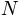is the number of pairs, this function is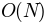for most of the heavy lifting, whereas calling cohere for each pair is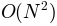. However, because of the caching, it is also more memory intensive, making 2 additional complex arrays with approximately the same number of elements as X. See `test/cohere_pairs_test.py` in the src tree for an example script that shows that this `cohere_pairs()` and `cohere()` give the same results for a given pair.

`psd()`
For information about the methods used to compute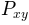,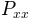and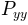.
`matplotlib.mlab.``complex_spectrum`(x, Fs=None, window=None, pad_to=None, sides=None)

Compute the complex-valued frequency spectrum of x. Data is padded to a length of pad_to and the windowing function window is applied to the signal.

Parameters: x : 1-D array or sequence Array or sequence containing the data Fs : scalar The sampling frequency (samples per time unit). It is used to calculate the Fourier frequencies, freqs, in cycles per time unit. The default value is 2. window : callable or ndarray A function or a vector of length NFFT. To create window vectors see `window_hanning()`, `window_none()`, `numpy.blackman()`, `numpy.hamming()`, `numpy.bartlett()`, `scipy.signal()`, `scipy.signal.get_window()`, etc. The default is `window_hanning()`. If a function is passed as the argument, it must take a data segment as an argument and return the windowed version of the segment. sides : [ ‘default’ | ‘onesided’ | ‘twosided’ ] Specifies which sides of the spectrum to return. Default gives the default behavior, which returns one-sided for real data and both for complex data. ‘onesided’ forces the return of a one-sided spectrum, while ‘twosided’ forces two-sided. pad_to : integer The number of points to which the data segment is padded when performing the FFT. While not increasing the actual resolution of the spectrum (the minimum distance between resolvable peaks), this can give more points in the plot, allowing for more detail. This corresponds to the n parameter in the call to fft(). The default is None, which sets pad_to equal to the length of the input signal (i.e. no padding). spectrum : 1-D array The values for the complex spectrum (complex valued) freqs : 1-D array The frequencies corresponding to the elements in spectrum

`magnitude_spectrum()`
`magnitude_spectrum()` returns the absolute value of this function.
`angle_spectrum()`
`angle_spectrum()` returns the angle of this function.
`phase_spectrum()`
`phase_spectrum()` returns the phase (unwrapped angle) of this function.
`specgram()`
`specgram()` can return the complex spectrum of segments within the signal.
`matplotlib.mlab.``contiguous_regions`(mask)

return a list of (ind0, ind1) such that mask[ind0:ind1].all() is True and we cover all such regions

`matplotlib.mlab.``cross_from_above`(x, threshold)

return the indices into x where x crosses some threshold from below, e.g., the i’s where:

```x[i-1]>threshold and x[i]<=threshold
```
`matplotlib.mlab.``cross_from_below`(x, threshold)

return the indices into x where x crosses some threshold from below, e.g., the i’s where:

```x[i-1]<threshold and x[i]>=threshold
```

Example code:

```import matplotlib.pyplot as plt

t = np.arange(0.0, 2.0, 0.1)
s = np.sin(2*np.pi*t)

fig = plt.figure()
ax.plot(t, s, '-o')
ax.axhline(0.5)
ax.axhline(-0.5)

ind = cross_from_below(s, 0.5)
ax.vlines(t[ind], -1, 1)

ind = cross_from_above(s, -0.5)
ax.vlines(t[ind], -1, 1)

plt.show()
```
`matplotlib.mlab.``csd`(x, y, NFFT=None, Fs=None, detrend=None, window=None, noverlap=None, pad_to=None, sides=None, scale_by_freq=None)

Compute the cross-spectral density.

Call signature:

```csd(x, y, NFFT=256, Fs=2, detrend=mlab.detrend_none,
sides='default', scale_by_freq=None)
```

The cross spectral densityby Welch’s average periodogram method. The vectors x and y are divided into NFFT length segments. Each segment is detrended by function detrend and windowed by function window. noverlap gives the length of the overlap between segments. The product of the direct FFTs of x and y are averaged over each segment to compute, with a scaling to correct for power loss due to windowing.

If len(x) < NFFT or len(y) < NFFT, they will be zero padded to NFFT.

Parameters: x, y : 1-D arrays or sequences Arrays or sequences containing the data Fs : scalar The sampling frequency (samples per time unit). It is used to calculate the Fourier frequencies, freqs, in cycles per time unit. The default value is 2. window : callable or ndarray A function or a vector of length NFFT. To create window vectors see `window_hanning()`, `window_none()`, `numpy.blackman()`, `numpy.hamming()`, `numpy.bartlett()`, `scipy.signal()`, `scipy.signal.get_window()`, etc. The default is `window_hanning()`. If a function is passed as the argument, it must take a data segment as an argument and return the windowed version of the segment. sides : [ ‘default’ | ‘onesided’ | ‘twosided’ ] Specifies which sides of the spectrum to return. Default gives the default behavior, which returns one-sided for real data and both for complex data. ‘onesided’ forces the return of a one-sided spectrum, while ‘twosided’ forces two-sided. pad_to : integer The number of points to which the data segment is padded when performing the FFT. This can be different from NFFT, which specifies the number of data points used. While not increasing the actual resolution of the spectrum (the minimum distance between resolvable peaks), this can give more points in the plot, allowing for more detail. This corresponds to the n parameter in the call to fft(). The default is None, which sets pad_to equal to NFFT NFFT : integer The number of data points used in each block for the FFT. A power 2 is most efficient. The default value is 256. This should NOT be used to get zero padding, or the scaling of the result will be incorrect. Use pad_to for this instead. detrend : {‘default’, ‘constant’, ‘mean’, ‘linear’, ‘none’} or callable The function applied to each segment before fft-ing, designed to remove the mean or linear trend. Unlike in MATLAB, where the detrend parameter is a vector, in matplotlib is it a function. The `pylab` module defines `detrend_none()`, `detrend_mean()`, and `detrend_linear()`, but you can use a custom function as well. You can also use a string to choose one of the functions. ‘default’, ‘constant’, and ‘mean’ call `detrend_mean()`. ‘linear’ calls `detrend_linear()`. ‘none’ calls `detrend_none()`. scale_by_freq : boolean, optional Specifies whether the resulting density values should be scaled by the scaling frequency, which gives density in units of Hz^-1. This allows for integration over the returned frequency values. The default is True for MATLAB compatibility. noverlap : integer The number of points of overlap between segments. The default value is 0 (no overlap). Pxy : 1-D array The values for the cross spectrum `P_{xy}` before scaling (real valued) freqs : 1-D array The frequencies corresponding to the elements in Pxy

`psd()`
`psd()` is the equivalent to setting y=x.

References

Bendat & Piersol – Random Data: Analysis and Measurement Procedures, John Wiley & Sons (1986)

`matplotlib.mlab.``csv2rec`(fname, comments='#', skiprows=0, checkrows=0, delimiter=', ', converterd=None, names=None, missing='', missingd=None, use_mrecords=False, dayfirst=False, yearfirst=False)

Load data from comma/space/tab delimited file in fname into a numpy record array and return the record array.

If names is None, a header row is required to automatically assign the recarray names. The headers will be lower cased, spaces will be converted to underscores, and illegal attribute name characters removed. If names is not None, it is a sequence of names to use for the column names. In this case, it is assumed there is no header row.

• fname: can be a filename or a file handle. Support for gzipped files is automatic, if the filename ends in ‘.gz’

• comments: the character used to indicate the start of a comment in the file, or None to switch off the removal of comments

• skiprows: is the number of rows from the top to skip

• checkrows: is the number of rows to check to validate the column data type. When set to zero all rows are validated.

• converterd: if not None, is a dictionary mapping column number or munged column name to a converter function.

• names: if not None, is a list of header names. In this case, no header will be read from the file

• missingd is a dictionary mapping munged column names to field values which signify that the field does not contain actual data and should be masked, e.g., ‘0000-00-00’ or ‘unused’

• missing: a string whose value signals a missing field regardless of the column it appears in

• use_mrecords: if True, return an mrecords.fromrecords record array if any of the data are missing

• dayfirst: default is False so that MM-DD-YY has precedence over DD-MM-YY. See http://labix.org/python-dateutil#head-b95ce2094d189a89f80f5ae52a05b4ab7b41af47 for further information.

• yearfirst: default is False so that MM-DD-YY has precedence over YY-MM-DD. See http://labix.org/python-dateutil#head-b95ce2094d189a89f80f5ae52a05b4ab7b41af47 for further information.

If no rows are found, None is returned – see `examples/loadrec.py`

`matplotlib.mlab.``csvformat_factory`(format)
`matplotlib.mlab.``demean`(x, axis=0)

Return x minus its mean along the specified axis.

Parameters: x : array or sequence Array or sequence containing the data Can have any dimensionality axis : integer The axis along which to take the mean. See numpy.mean for a description of this argument.

`delinear()`

`denone()`
`delinear()` and `denone()` are other detrend algorithms.
`detrend_mean()`
This function is the same as `detrend_mean()` except for the default axis.
`matplotlib.mlab.``detrend`(x, key=None, axis=None)

Return x with its trend removed.

Parameters: x : array or sequence Array or sequence containing the data. key : [ ‘default’ | ‘constant’ | ‘mean’ | ‘linear’ | ‘none’] or function Specifies the detrend algorithm to use. ‘default’ is ‘mean’, which is the same as `detrend_mean()`. ‘constant’ is the same. ‘linear’ is the same as `detrend_linear()`. ‘none’ is the same as `detrend_none()`. The default is ‘mean’. See the corresponding functions for more details regarding the algorithms. Can also be a function that carries out the detrend operation. axis : integer The axis along which to do the detrending.

`detrend_mean()`
`detrend_mean()` implements the ‘mean’ algorithm.
`detrend_linear()`
`detrend_linear()` implements the ‘linear’ algorithm.
`detrend_none()`
`detrend_none()` implements the ‘none’ algorithm.
`matplotlib.mlab.``detrend_linear`(y)

Return x minus best fit line; ‘linear’ detrending.

Parameters: y : 0-D or 1-D array or sequence Array or sequence containing the data axis : integer The axis along which to take the mean. See numpy.mean for a description of this argument.

`delinear()`
This function is the same as `delinear()` except for the default axis.

`detrend_mean()`

`detrend_none()`
`detrend_mean()` and `detrend_none()` are other detrend algorithms.
`detrend()`
`detrend()` is a wrapper around all the detrend algorithms.
`matplotlib.mlab.``detrend_mean`(x, axis=None)

Return x minus the mean(x).

Parameters: x : array or sequence Array or sequence containing the data Can have any dimensionality axis : integer The axis along which to take the mean. See numpy.mean for a description of this argument.

`demean()`
This function is the same as `demean()` except for the default axis.

`detrend_linear()`

`detrend_none()`
`detrend_linear()` and `detrend_none()` are other detrend algorithms.
`detrend()`
`detrend()` is a wrapper around all the detrend algorithms.
`matplotlib.mlab.``detrend_none`(x, axis=None)

Return x: no detrending.

Parameters: x : any object An object containing the data axis : integer This parameter is ignored. It is included for compatibility with detrend_mean

`denone()`
This function is the same as `denone()` except for the default axis, which has no effect.

`detrend_mean()`

`detrend_linear()`
`detrend_mean()` and `detrend_linear()` are other detrend algorithms.
`detrend()`
`detrend()` is a wrapper around all the detrend algorithms.
`matplotlib.mlab.``dist`(x, y)

Return the distance between two points.

`matplotlib.mlab.``dist_point_to_segment`(p, s0, s1)

Get the distance of a point to a segment.

p, s0, s1 are xy sequences

This algorithm from http://geomalgorithms.com/a02-_lines.html

`matplotlib.mlab.``distances_along_curve`(X)

Computes the distance between a set of successive points in N dimensions.

Where X is an M x N array or matrix. The distances between successive rows is computed. Distance is the standard Euclidean distance.

`matplotlib.mlab.``donothing_callback`(*args)
`matplotlib.mlab.``entropy`(y, bins)

Return the entropy of the data in y in units of nat.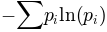where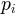is the probability of observing y in the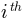bin of bins. bins can be a number of bins or a range of bins; see `numpy.histogram()`.

Compare S with analytic calculation for a Gaussian:

```x = mu + sigma * randn(200000)
Sanalytic = 0.5 * ( 1.0 + log(2*pi*sigma**2.0) )
```
`matplotlib.mlab.``exp_safe`(x)

Compute exponentials which safely underflow to zero.

Slow, but convenient to use. Note that numpy provides proper floating point exception handling with access to the underlying hardware.

`matplotlib.mlab.``fftsurr`(x, detrend=<function detrend_none>, window=<function window_none>)

Compute an FFT phase randomized surrogate of x.

`matplotlib.mlab.``find`(condition)

Return the indices where ravel(condition) is true

`matplotlib.mlab.``frange`([start, ]stop[, step, keywords]) → array of floats

Return a numpy ndarray containing a progression of floats. Similar to `numpy.arange()`, but defaults to a closed interval.

`frange(x0, x1)` returns `[x0, x0+1, x0+2, ..., x1]`; start defaults to 0, and the endpoint is included. This behavior is different from that of `range()` and `numpy.arange()`. This is deliberate, since `frange()` will probably be more useful for generating lists of points for function evaluation, and endpoints are often desired in this use. The usual behavior of `range()` can be obtained by setting the keyword closed = 0, in this case, `frange()` basically becomes :func:numpy.arange`.

When step is given, it specifies the increment (or decrement). All arguments can be floating point numbers.

`frange(x0,x1,d)` returns `[x0,x0+d,x0+2d,...,xfin]` where xfin <= x1.

`frange()` can also be called with the keyword npts. This sets the number of points the list should contain (and overrides the value step might have been given). `numpy.arange()` doesn’t offer this option.

Examples:

```>>> frange(3)
array([ 0.,  1.,  2.,  3.])
>>> frange(3,closed=0)
array([ 0.,  1.,  2.])
>>> frange(1,6,2)
array([1, 3, 5])   or 1,3,5,7, depending on floating point vagueries
>>> frange(1,6.5,npts=5)
array([ 1.   ,  2.375,  3.75 ,  5.125,  6.5  ])
```
`matplotlib.mlab.``get_formatd`(r, formatd=None)

build a formatd guaranteed to have a key for every dtype name

`matplotlib.mlab.``get_sparse_matrix`(M, N, frac=0.1)

Return a M x N sparse matrix with frac elements randomly filled.

`matplotlib.mlab.``get_xyz_where`(Z, Cond)

Z and Cond are M x N matrices. Z are data and Cond is a boolean matrix where some condition is satisfied. Return value is (x, y, z) where x and y are the indices into Z and z are the values of Z at those indices. x, y, and z are 1D arrays.

`matplotlib.mlab.``griddata`(x, y, z, xi, yi, interp='nn')

Interpolates from a nonuniformly spaced grid to some other grid.

Fits a surface of the form z = f(`x`, `y`) to the data in the (usually) nonuniformly spaced vectors (`x`, `y`, `z`), then interpolates this surface at the points specified by (`xi`, `yi`) to produce `zi`.

Parameters: x, y, z : 1d array_like Coordinates of grid points to interpolate from. xi, yi : 1d or 2d array_like Coordinates of grid points to interpolate to. interp : string key from {‘nn’, ‘linear’} Interpolation algorithm, either ‘nn’ for natural neighbor, or ‘linear’ for linear interpolation. 2d float array Array of values interpolated at (`xi`, `yi`) points. Array will be masked is any of (`xi`, `yi`) are outside the convex hull of (`x`, `y`).

Notes

If `interp` is ‘nn’ (the default), uses natural neighbor interpolation based on Delaunay triangulation. This option is only available if the mpl_toolkits.natgrid module is installed. This can be downloaded from https://github.com/matplotlib/natgrid. The (`xi`, `yi`) grid must be regular and monotonically increasing in this case.

If `interp` is ‘linear’, linear interpolation is used via matplotlib.tri.LinearTriInterpolator.

Instead of using `griddata`, more flexible functionality and other interpolation options are available using a matplotlib.tri.Triangulation and a matplotlib.tri.TriInterpolator.

`matplotlib.mlab.``identity`(n, rank=2, dtype='l', typecode=None)

Returns the identity matrix of shape (n, n, ..., n) (rank r).

For ranks higher than 2, this object is simply a multi-index Kronecker delta:

```                    /  1  if i0=i1=...=iR,
id[i0,i1,...,iR] = -|
\  0  otherwise.
```

Optionally a dtype (or typecode) may be given (it defaults to ‘l’).

Since rank defaults to 2, this function behaves in the default case (when only n is given) like `numpy.identity(n)` – but surprisingly, it is much faster.

`matplotlib.mlab.``inside_poly`(points, verts)

points is a sequence of x, y points. verts is a sequence of x, y vertices of a polygon.

Return value is a sequence of indices into points for the points that are inside the polygon.

`matplotlib.mlab.``is_closed_polygon`(X)

Tests whether first and last object in a sequence are the same. These are presumably coordinates on a polygonal curve, in which case this function tests if that curve is closed.

`matplotlib.mlab.``ispower2`(n)

Returns the log base 2 of n if n is a power of 2, zero otherwise.

Note the potential ambiguity if n == 1: 2**0 == 1, interpret accordingly.

`matplotlib.mlab.``isvector`(X)

Like the MATLAB function with the same name, returns True if the supplied numpy array or matrix X looks like a vector, meaning it has a one non-singleton axis (i.e., it can have multiple axes, but all must have length 1, except for one of them).

If you just want to see if the array has 1 axis, use X.ndim == 1.

`matplotlib.mlab.``l1norm`(a)

Return the l1 norm of a, flattened out.

Implemented as a separate function (not a call to `norm()` for speed).

`matplotlib.mlab.``l2norm`(a)

Return the l2 norm of a, flattened out.

Implemented as a separate function (not a call to `norm()` for speed).

`matplotlib.mlab.``less_simple_linear_interpolation`(x, y, xi, extrap=False)

This function provides simple (but somewhat less so than `cbook.simple_linear_interpolation()`) linear interpolation. `simple_linear_interpolation()` will give a list of point between a start and an end, while this does true linear interpolation at an arbitrary set of points.

This is very inefficient linear interpolation meant to be used only for a small number of points in relatively non-intensive use cases. For real linear interpolation, use scipy.

`matplotlib.mlab.``log2`(x, ln2=0.6931471805599453)

Return the log(x) in base 2.

This is a _slow_ function but which is guaranteed to return the correct integer value if the input is an integer exact power of 2.

`matplotlib.mlab.``logspace`(xmin, xmax, N)

Return N values logarithmically spaced between xmin and xmax.

`matplotlib.mlab.``longest_contiguous_ones`(x)

Return the indices of the longest stretch of contiguous ones in x, assuming x is a vector of zeros and ones. If there are two equally long stretches, pick the first.

`matplotlib.mlab.``longest_ones`(x)

alias for longest_contiguous_ones

`matplotlib.mlab.``magnitude_spectrum`(x, Fs=None, window=None, pad_to=None, sides=None)

Compute the magnitude (absolute value) of the frequency spectrum of x. Data is padded to a length of pad_to and the windowing function window is applied to the signal.

Parameters: x : 1-D array or sequence Array or sequence containing the data Fs : scalar The sampling frequency (samples per time unit). It is used to calculate the Fourier frequencies, freqs, in cycles per time unit. The default value is 2. window : callable or ndarray A function or a vector of length NFFT. To create window vectors see `window_hanning()`, `window_none()`, `numpy.blackman()`, `numpy.hamming()`, `numpy.bartlett()`, `scipy.signal()`, `scipy.signal.get_window()`, etc. The default is `window_hanning()`. If a function is passed as the argument, it must take a data segment as an argument and return the windowed version of the segment. sides : [ ‘default’ | ‘onesided’ | ‘twosided’ ] Specifies which sides of the spectrum to return. Default gives the default behavior, which returns one-sided for real data and both for complex data. ‘onesided’ forces the return of a one-sided spectrum, while ‘twosided’ forces two-sided. pad_to : integer The number of points to which the data segment is padded when performing the FFT. While not increasing the actual resolution of the spectrum (the minimum distance between resolvable peaks), this can give more points in the plot, allowing for more detail. This corresponds to the n parameter in the call to fft(). The default is None, which sets pad_to equal to the length of the input signal (i.e. no padding). spectrum : 1-D array The values for the magnitude spectrum (real valued) freqs : 1-D array The frequencies corresponding to the elements in spectrum

`psd()`
`psd()` returns the power spectral density.
`complex_spectrum()`
This function returns the absolute value of `complex_spectrum()`.
`angle_spectrum()`
`angle_spectrum()` returns the angles of the corresponding frequencies.
`phase_spectrum()`
`phase_spectrum()` returns the phase (unwrapped angle) of the corresponding frequencies.
`specgram()`
`specgram()` can return the magnitude spectrum of segments within the signal.
`matplotlib.mlab.``movavg`(x, n)

Compute the len(n) moving average of x.

`matplotlib.mlab.``norm_flat`(a, p=2)

norm(a,p=2) -> l-p norm of a.flat

Return the l-p norm of a, considered as a flat array. This is NOT a true matrix norm, since arrays of arbitrary rank are always flattened.

p can be a number or the string ‘Infinity’ to get the L-infinity norm.

`matplotlib.mlab.``normpdf`(x, *args)

Return the normal pdf evaluated at x; args provides mu, sigma

`matplotlib.mlab.``offset_line`(y, yerr)

Offsets an array y by +/- an error and returns a tuple (y - err, y + err).

The error term can be:

• A scalar. In this case, the returned tuple is obvious.

• A vector of the same length as y. The quantities y +/- err are computed component-wise.

• A tuple of length 2. In this case, yerr is the error below y and yerr is error above y. For example:

```from pylab import *
x = linspace(0, 2*pi, num=100, endpoint=True)
y = sin(x)
y_minus, y_plus = mlab.offset_line(y, 0.1)
plot(x, y)
fill_between(x, ym, y2=yp)
show()
```
`matplotlib.mlab.``path_length`(X)

Computes the distance travelled along a polygonal curve in N dimensions.

Where X is an M x N array or matrix. Returns an array of length M consisting of the distance along the curve at each point (i.e., the rows of X).

`matplotlib.mlab.``phase_spectrum`(x, Fs=None, window=None, pad_to=None, sides=None)

Compute the phase of the frequency spectrum (unwrapped angle spectrum) of x. Data is padded to a length of pad_to and the windowing function window is applied to the signal.

Parameters: x : 1-D array or sequence Array or sequence containing the data Fs : scalar The sampling frequency (samples per time unit). It is used to calculate the Fourier frequencies, freqs, in cycles per time unit. The default value is 2. window : callable or ndarray A function or a vector of length NFFT. To create window vectors see `window_hanning()`, `window_none()`, `numpy.blackman()`, `numpy.hamming()`, `numpy.bartlett()`, `scipy.signal()`, `scipy.signal.get_window()`, etc. The default is `window_hanning()`. If a function is passed as the argument, it must take a data segment as an argument and return the windowed version of the segment. sides : [ ‘default’ | ‘onesided’ | ‘twosided’ ] Specifies which sides of the spectrum to return. Default gives the default behavior, which returns one-sided for real data and both for complex data. ‘onesided’ forces the return of a one-sided spectrum, while ‘twosided’ forces two-sided. pad_to : integer The number of points to which the data segment is padded when performing the FFT. While not increasing the actual resolution of the spectrum (the minimum distance between resolvable peaks), this can give more points in the plot, allowing for more detail. This corresponds to the n parameter in the call to fft(). The default is None, which sets pad_to equal to the length of the input signal (i.e. no padding). spectrum : 1-D array The values for the phase spectrum in radians (real valued) freqs : 1-D array The frequencies corresponding to the elements in spectrum

`complex_spectrum()`
This function returns the angle value of `complex_spectrum()`.
`magnitude_spectrum()`
`magnitude_spectrum()` returns the magnitudes of the corresponding frequencies.
`angle_spectrum()`
`angle_spectrum()` returns the wrapped version of this function.
`specgram()`
`specgram()` can return the phase spectrum of segments within the signal.
`matplotlib.mlab.``poly_below`(xmin, xs, ys)

Given a sequence of xs and ys, return the vertices of a polygon that has a horizontal base at xmin and an upper bound at the ys. xmin is a scalar.

Intended for use with `matplotlib.axes.Axes.fill()`, e.g.,:

```xv, yv = poly_below(0, x, y)
ax.fill(xv, yv)
```
`matplotlib.mlab.``poly_between`(x, ylower, yupper)

Given a sequence of x, ylower and yupper, return the polygon that fills the regions between them. ylower or yupper can be scalar or iterable. If they are iterable, they must be equal in length to x.

Return value is x, y arrays for use with `matplotlib.axes.Axes.fill()`.

`matplotlib.mlab.``prctile`(x, p=(0.0, 25.0, 50.0, 75.0, 100.0))

Return the percentiles of x. p can either be a sequence of percentile values or a scalar. If p is a sequence, the ith element of the return sequence is the p*(i)-th percentile of *x. If p is a scalar, the largest value of x less than or equal to the p percentage point in the sequence is returned.

`matplotlib.mlab.``prctile_rank`(x, p)

Return the rank for each element in x, return the rank 0..len(p). e.g., if p = (25, 50, 75), the return value will be a len(x) array with values in [0,1,2,3] where 0 indicates the value is less than the 25th percentile, 1 indicates the value is >= the 25th and < 50th percentile, ... and 3 indicates the value is above the 75th percentile cutoff.

p is either an array of percentiles in [0..100] or a scalar which indicates how many quantiles of data you want ranked.

`matplotlib.mlab.``psd`(x, NFFT=None, Fs=None, detrend=None, window=None, noverlap=None, pad_to=None, sides=None, scale_by_freq=None)

Compute the power spectral density.

Call signature:

```psd(x, NFFT=256, Fs=2, detrend=mlab.detrend_none,
sides='default', scale_by_freq=None)
```

The power spectral densityby Welch’s average periodogram method. The vector x is divided into NFFT length segments. Each segment is detrended by function detrend and windowed by function window. noverlap gives the length of the overlap between segments. The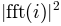of each segment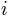are averaged to compute.

If len(x) < NFFT, it will be zero padded to NFFT.

Parameters: x : 1-D array or sequence Array or sequence containing the data Fs : scalar The sampling frequency (samples per time unit). It is used to calculate the Fourier frequencies, freqs, in cycles per time unit. The default value is 2. window : callable or ndarray A function or a vector of length NFFT. To create window vectors see `window_hanning()`, `window_none()`, `numpy.blackman()`, `numpy.hamming()`, `numpy.bartlett()`, `scipy.signal()`, `scipy.signal.get_window()`, etc. The default is `window_hanning()`. If a function is passed as the argument, it must take a data segment as an argument and return the windowed version of the segment. sides : [ ‘default’ | ‘onesided’ | ‘twosided’ ] Specifies which sides of the spectrum to return. Default gives the default behavior, which returns one-sided for real data and both for complex data. ‘onesided’ forces the return of a one-sided spectrum, while ‘twosided’ forces two-sided. pad_to : integer The number of points to which the data segment is padded when performing the FFT. This can be different from NFFT, which specifies the number of data points used. While not increasing the actual resolution of the spectrum (the minimum distance between resolvable peaks), this can give more points in the plot, allowing for more detail. This corresponds to the n parameter in the call to fft(). The default is None, which sets pad_to equal to NFFT NFFT : integer The number of data points used in each block for the FFT. A power 2 is most efficient. The default value is 256. This should NOT be used to get zero padding, or the scaling of the result will be incorrect. Use pad_to for this instead. detrend : {‘default’, ‘constant’, ‘mean’, ‘linear’, ‘none’} or callable The function applied to each segment before fft-ing, designed to remove the mean or linear trend. Unlike in MATLAB, where the detrend parameter is a vector, in matplotlib is it a function. The `pylab` module defines `detrend_none()`, `detrend_mean()`, and `detrend_linear()`, but you can use a custom function as well. You can also use a string to choose one of the functions. ‘default’, ‘constant’, and ‘mean’ call `detrend_mean()`. ‘linear’ calls `detrend_linear()`. ‘none’ calls `detrend_none()`. scale_by_freq : boolean, optional Specifies whether the resulting density values should be scaled by the scaling frequency, which gives density in units of Hz^-1. This allows for integration over the returned frequency values. The default is True for MATLAB compatibility. noverlap : integer The number of points of overlap between segments. The default value is 0 (no overlap). Pxx : 1-D array The values for the power spectrum `P_{xx}` (real valued) freqs : 1-D array The frequencies corresponding to the elements in Pxx

`specgram()`
`specgram()` differs in the default overlap; in not returning the mean of the segment periodograms; and in returning the times of the segments.
`magnitude_spectrum()`
`magnitude_spectrum()` returns the magnitude spectrum.
`csd()`
`csd()` returns the spectral density between two signals.

References

Bendat & Piersol – Random Data: Analysis and Measurement Procedures, John Wiley & Sons (1986)

`matplotlib.mlab.``quad2cubic`(q0x, q0y, q1x, q1y, q2x, q2y)

Converts a quadratic Bezier curve to a cubic approximation.

The inputs are the x and y coordinates of the three control points of a quadratic curve, and the output is a tuple of x and y coordinates of the four control points of the cubic curve.

`matplotlib.mlab.``rec2csv`(r, fname, delimiter=', ', formatd=None, missing='', missingd=None, withheader=True)

Save the data from numpy recarray r into a comma-/space-/tab-delimited file. The record array dtype names will be used for column headers.

fname: can be a filename or a file handle. Support for gzipped
files is automatic, if the filename ends in ‘.gz’
withheader: if withheader is False, do not write the attribute
names in the first row

for formatd type FormatFloat, we override the precision to store full precision floats in the CSV file

`csv2rec()`
For information about missing and missingd, which can be used to fill in masked values into your CSV file.
`matplotlib.mlab.``rec2txt`(r, header=None, padding=3, precision=3, fields=None)

Returns a textual representation of a record array.

Parameters: r: numpy recarray header: list column headers padding: space between each column precision: number of decimal places to use for floats. Set to an integer to apply to all floats. Set to a list of integers to apply precision individually. Precision for non-floats is simply ignored. fields : list If not None, a list of field names to print. fields can be a list of strings like [‘field1’, ‘field2’] or a single comma separated string like ‘field1,field2’

Examples

For `precision=[0,2,3]`, the output is

```ID    Price   Return
ABC   12.54    0.234
XYZ    6.32   -0.076
```
`matplotlib.mlab.``rec_append_fields`(rec, names, arrs, dtypes=None)

Return a new record array with field names populated with data from arrays in arrs. If appending a single field, then names, arrs and dtypes do not have to be lists. They can just be the values themselves.

`matplotlib.mlab.``rec_drop_fields`(rec, names)

Return a new numpy record array with fields in names dropped.

`matplotlib.mlab.``rec_groupby`(r, groupby, stats)

r is a numpy record array

groupby is a sequence of record array attribute names that together form the grouping key. e.g., (‘date’, ‘productcode’)

stats is a sequence of (attr, func, outname) tuples which will call `x = func(attr)` and assign x to the record array output with attribute outname. For example:

```stats = ( ('sales', len, 'numsales'), ('sales', np.mean, 'avgsale') )
```

Return record array has dtype names for each attribute name in the groupby argument, with the associated group values, and for each outname name in the stats argument, with the associated stat summary output.

`matplotlib.mlab.``rec_join`(key, r1, r2, jointype='inner', defaults=None, r1postfix='1', r2postfix='2')

Join record arrays r1 and r2 on key; key is a tuple of field names – if key is a string it is assumed to be a single attribute name. If r1 and r2 have equal values on all the keys in the key tuple, then their fields will be merged into a new record array containing the intersection of the fields of r1 and r2.

r1 (also r2) must not have any duplicate keys.

The jointype keyword can be ‘inner’, ‘outer’, ‘leftouter’. To do a rightouter join just reverse r1 and r2.

The defaults keyword is a dictionary filled with `{column_name:default_value}` pairs.

The keywords r1postfix and r2postfix are postfixed to column names (other than keys) that are both in r1 and r2.

`matplotlib.mlab.``rec_keep_fields`(rec, names)

Return a new numpy record array with only fields listed in names

`matplotlib.mlab.``rec_summarize`(r, summaryfuncs)

r is a numpy record array

summaryfuncs is a list of (attr, func, outname) tuples which will apply func to the array r*[attr] and assign the output to a new attribute name *outname. The returned record array is identical to r, with extra arrays for each element in summaryfuncs.

`matplotlib.mlab.``recs_join`(key, name, recs, jointype='outer', missing=0.0, postfixes=None)

Join a sequence of record arrays on single column key.

This function only joins a single column of the multiple record arrays

key
is the column name that acts as a key
name
is the name of the column that we want to join
recs
is a list of record arrays to join
jointype
is a string ‘inner’ or ‘outer’
missing
is what any missing field is replaced by
postfixes
if not None, a len recs sequence of postfixes

returns a record array with columns [rowkey, name0, name1, ... namen-1]. or if postfixes [PF0, PF1, ..., PFN-1] are supplied, [rowkey, namePF0, namePF1, ... namePFN-1].

Example:

```r = recs_join("date", "close", recs=[r0, r1], missing=0.)
```
`matplotlib.mlab.``rk4`(derivs, y0, t)

Integrate 1D or ND system of ODEs using 4-th order Runge-Kutta. This is a toy implementation which may be useful if you find yourself stranded on a system w/o scipy. Otherwise use `scipy.integrate()`.

Parameters: y0 initial state vector t sample times derivs returns the derivative of the system and has the signature `dy = derivs(yi, ti)`

Examples

A 2D system:

```def derivs6(x,t):
d1 =  x + 2*x
d2 =  -3*x + 4*x
return (d1, d2)
dt = 0.0005
t = arange(0.0, 2.0, dt)
y0 = (1,2)
yout = rk4(derivs6, y0, t)
```

A 1D system:

```alpha = 2
def derivs(x,t):
return -alpha*x + exp(-t)

y0 = 1
yout = rk4(derivs, y0, t)
```

If you have access to scipy, you should probably be using the scipy.integrate tools rather than this function.

`matplotlib.mlab.``rms_flat`(a)

Return the root mean square of all the elements of a, flattened out.

`matplotlib.mlab.``safe_isinf`(x)

`numpy.isinf()` for arbitrary types

`matplotlib.mlab.``safe_isnan`(x)

`numpy.isnan()` for arbitrary types

`matplotlib.mlab.``segments_intersect`(s1, s2)

Return True if s1 and s2 intersect. s1 and s2 are defined as:

```s1: (x1, y1), (x2, y2)
s2: (x3, y3), (x4, y4)
```
`matplotlib.mlab.``slopes`(x, y)

`slopes()` calculates the slope y‘(x)

The slope is estimated using the slope obtained from that of a parabola through any three consecutive points.

This method should be superior to that described in the appendix of A CONSISTENTLY WELL BEHAVED METHOD OF INTERPOLATION by Russel W. Stineman (Creative Computing July 1980) in at least one aspect:

Circles for interpolation demand a known aspect ratio between x- and y-values. For many functions, however, the abscissa are given in different dimensions, so an aspect ratio is completely arbitrary.

The parabola method gives very similar results to the circle method for most regular cases but behaves much better in special cases.

Norbert Nemec, Institute of Theoretical Physics, University or Regensburg, April 2006 Norbert.Nemec at physik.uni-regensburg.de

(inspired by a original implementation by Halldor Bjornsson, Icelandic Meteorological Office, March 2006 halldor at vedur.is)

`matplotlib.mlab.``specgram`(x, NFFT=None, Fs=None, detrend=None, window=None, noverlap=None, pad_to=None, sides=None, scale_by_freq=None, mode=None)

Compute a spectrogram.

Compute and plot a spectrogram of data in x. Data are split into NFFT length segments and the spectrum of each section is computed. The windowing function window is applied to each segment, and the amount of overlap of each segment is specified with noverlap.

Parameters: x : array_like 1-D array or sequence. Fs : scalar The sampling frequency (samples per time unit). It is used to calculate the Fourier frequencies, freqs, in cycles per time unit. The default value is 2. window : callable or ndarray A function or a vector of length NFFT. To create window vectors see `window_hanning()`, `window_none()`, `numpy.blackman()`, `numpy.hamming()`, `numpy.bartlett()`, `scipy.signal()`, `scipy.signal.get_window()`, etc. The default is `window_hanning()`. If a function is passed as the argument, it must take a data segment as an argument and return the windowed version of the segment. sides : [ ‘default’ | ‘onesided’ | ‘twosided’ ] Specifies which sides of the spectrum to return. Default gives the default behavior, which returns one-sided for real data and both for complex data. ‘onesided’ forces the return of a one-sided spectrum, while ‘twosided’ forces two-sided. pad_to : integer The number of points to which the data segment is padded when performing the FFT. This can be different from NFFT, which specifies the number of data points used. While not increasing the actual resolution of the spectrum (the minimum distance between resolvable peaks), this can give more points in the plot, allowing for more detail. This corresponds to the n parameter in the call to fft(). The default is None, which sets pad_to equal to NFFT NFFT : integer The number of data points used in each block for the FFT. A power 2 is most efficient. The default value is 256. This should NOT be used to get zero padding, or the scaling of the result will be incorrect. Use pad_to for this instead. detrend : {‘default’, ‘constant’, ‘mean’, ‘linear’, ‘none’} or callable The function applied to each segment before fft-ing, designed to remove the mean or linear trend. Unlike in MATLAB, where the detrend parameter is a vector, in matplotlib is it a function. The `pylab` module defines `detrend_none()`, `detrend_mean()`, and `detrend_linear()`, but you can use a custom function as well. You can also use a string to choose one of the functions. ‘default’, ‘constant’, and ‘mean’ call `detrend_mean()`. ‘linear’ calls `detrend_linear()`. ‘none’ calls `detrend_none()`. scale_by_freq : boolean, optional Specifies whether the resulting density values should be scaled by the scaling frequency, which gives density in units of Hz^-1. This allows for integration over the returned frequency values. The default is True for MATLAB compatibility. noverlap : int, optional The number of points of overlap between blocks. The default value is 128. mode : str, optional What sort of spectrum to use, default is ‘psd’. ‘psd’ Returns the power spectral density. ‘complex’ Returns the complex-valued frequency spectrum. ‘magnitude’ Returns the magnitude spectrum. ‘angle’ Returns the phase spectrum without unwrapping. ‘phase’ Returns the phase spectrum with unwrapping. spectrum : array_like 2-D array, columns are the periodograms of successive segments. freqs : array_like 1-D array, frequencies corresponding to the rows in spectrum. t : array_like 1-D array, the times corresponding to midpoints of segments (i.e the columns in spectrum).

`psd`
differs in the overlap and in the return values.
`complex_spectrum`
similar, but with complex valued frequencies.
`magnitude_spectrum`
similar single segment when mode is ‘magnitude’.
`angle_spectrum`
similar to single segment when mode is ‘angle’.
`phase_spectrum`
similar to single segment when mode is ‘phase’.

Notes

detrend and scale_by_freq only apply when mode is set to ‘psd’.

`matplotlib.mlab.``stineman_interp`(xi, x, y, yp=None)

Given data vectors x and y, the slope vector yp and a new abscissa vector xi, the function `stineman_interp()` uses Stineman interpolation to calculate a vector yi corresponding to xi.

Here’s an example that generates a coarse sine curve, then interpolates over a finer abscissa:

```x = linspace(0,2*pi,20);  y = sin(x); yp = cos(x)
xi = linspace(0,2*pi,40);
yi = stineman_interp(xi,x,y,yp);
plot(x,y,'o',xi,yi)
```

The interpolation method is described in the article A CONSISTENTLY WELL BEHAVED METHOD OF INTERPOLATION by Russell W. Stineman. The article appeared in the July 1980 issue of Creative Computing with a note from the editor stating that while they were:

not an academic journal but once in a while something serious and original comes in adding that this was “apparently a real solution” to a well known problem.

For yp = None, the routine automatically determines the slopes using the `slopes()` routine.

x is assumed to be sorted in increasing order.

For values `xi[j] < x` or `xi[j] > x[-1]`, the routine tries an extrapolation. The relevance of the data obtained from this, of course, is questionable...

Original implementation by Halldor Bjornsson, Icelandic Meteorolocial Office, March 2006 halldor at vedur.is

Completely reworked and optimized for Python by Norbert Nemec, Institute of Theoretical Physics, University or Regensburg, April 2006 Norbert.Nemec at physik.uni-regensburg.de

`matplotlib.mlab.``stride_repeat`(x, n, axis=0)

Repeat the values in an array in a memory-efficient manner. Array x is stacked vertically n times.

Warning

It is not safe to write to the output array. Multiple elements may point to the same piece of memory, so modifying one value may change others.

Parameters: x : 1D array or sequence Array or sequence containing the data. n : integer The number of time to repeat the array. axis : integer The axis along which the data will run.

References

stackoverflow: Repeat NumPy array without replicating data?

`matplotlib.mlab.``stride_windows`(x, n, noverlap=None, axis=0)

Get all windows of x with length n as a single array, using strides to avoid data duplication.

Warning

It is not safe to write to the output array. Multiple elements may point to the same piece of memory, so modifying one value may change others.

Parameters: x : 1D array or sequence Array or sequence containing the data. n : integer The number of data points in each window. noverlap : integer The overlap between adjacent windows. Default is 0 (no overlap) axis : integer The axis along which the windows will run.

References

`matplotlib.mlab.``vector_lengths`(X, P=2.0, axis=None)

Finds the length of a set of vectors in n dimensions. This is like the `numpy.norm()` function for vectors, but has the ability to work over a particular axis of the supplied array or matrix.

Computes `(sum((x_i)^P))^(1/P)` for each `{x_i}` being the elements of X along the given axis. If axis is None, compute over all elements of X.

`matplotlib.mlab.``window_hanning`(x)

Return x times the hanning window of len(x).

`window_none()`
`window_none()` is another window algorithm.
`matplotlib.mlab.``window_none`(x)
`window_hanning()`
`window_hanning()` is another window algorithm.